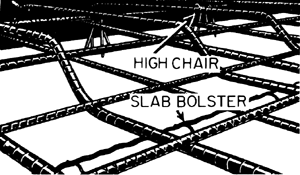# reinforcement ratio

## reinforcement ratioAt any section of a reinforced concrete structural member, the ratio of the effective area of the reinforcement to the effective area of the concrete.
McGraw-Hill Dictionary of Architecture and Construction. Copyright © 2003 by McGraw-Hill Companies, Inc.
References in periodicals archive ?
If the change in the response ratio is greater than a change in the reinforcement ratio, the value of a is greater than 1.
where: [E.sub.s]-modulus of elasticity of steel reinforcement; [tau]-shear stress; [[rho].sub.f]-longitudinal FRP reinforcement ratio.
in which [p.sub.w] is the web reinforcement ratio for the tensile reinforcement [A.sub.st]/([b.sub.w]d), [p.sub.cw] is the web reinforcement ratio for the compressive reinforcement [A.sub.sc]/([b.sub.w]d) and [[epsilon].sub.cs] is the design shrinkage strain of the concrete at the time the total or incremental deflection is required.
The increase of reinforcement ratio values from [micro]=1.1% up to [micro]=2.3%, for the elements made of concrete class grade C20/25 concrete reduce the time of initiation of first crack from 200 till 100 days.
where [M.sub.a] is the acting moment in a cross-section, [[rho].sub.c.eff] is a ratio of the effective area in tension, and [[rho].sub.eq] is the equivalent reinforcement ratio.
In addition, we can find that the ductility index increases as the reinforcement ratio is reduced by comparing the results of B-GR-0-T and B-MS-0-T.
Keeping the reinforcement ratio and 20 cm stirrup interval fixed, the change in abutment backwall cracking with the change in diameter and interval of horizontal crack-control reinforcement was evaluated.
 carried out triaxial unconsolidated undrained tests on coconut shell fiber-reinforced soft clay, and the tests results showed that 1% is the optimal fiber reinforcement ratio, and the reinforcement effect is more obvious under high confining pressure.
In Beam B2, the stirrups were arranged at 250 mm centres, corresponding to a reinforcement ratio of 0.4%.
It is well known that reinforced concrete strength, ductility and energy dissipation capacity increases with increase in confining reinforcement ratio. Thus concrete fabricated from low strength concrete and improved confinement will exhibit more ductility, therefore, suitable for use anywhere specially earthquake prone areas.
This paper presents results of 18 tests on one-way reinforced concrete slabs submitted to point loads, in which were varied the loading position (a), the slabs' width and the flexural reinforcement ratio in the transverse direction ([[rho].sub.[gamma]]).
Site: Follow: Share:
Open / Close